SEARCH SITE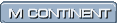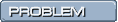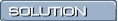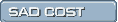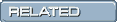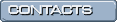AUTOMATED CAS BUG LISTS GENERATION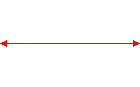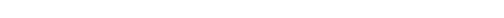This site is dedicated to my Teachers,
A., A., A., G., K., M., R., R., R. and S.,
and their attempts to tame ComplexityOur patent pending methodologies, LIFT and CYCLE, insure that your business objectives will not be sacrificed to the whims or oversights of CAS architects and developers.

The most important question is how will a computer algebra system satisfy your prospects, make you money and serve your needs.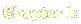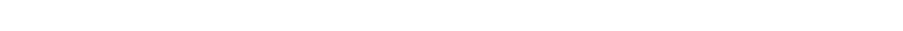print: KERNEL FAILURE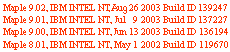> print(singular);     # Standard Worksheet

Kernel Failure

MUST BE: proc (expr::{algebraic, ...
HELP: TYPOSGuassian ojbects elemements sytem nfinity
coorindates docecahedron knowns inteter
specifiy parition revoltion satisifies electic errror commmand  transormation fuction....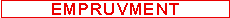evalf: KERNEL FAILURE>evalf(Int(sqrt(z)*BesselJ(0,z),z=0..infinity));

Kernel Failure

MUST BE:  0.4779887975
.
int: SPURIOUS VARIABLE> int((1-z)^z,z=0..1);

1/(1+z)

MUST BE:
int((1-z)^z, z = (0 .. 1))
limit: SPURIOUS RANGE> limit(sin(z)^2+cos(z)^2, z = infinity);

0 .. 2

MUST BE:  1
limit: SPURIOUS undefined> [limit(tan(z)*cot(z),z = infinity),
limit(simplify(tan(z)*cot(z)),z = infinity)];
[undefined, 1]

MUST BE:  [1, 1]
limit: SPURIOUS RANGE> limit(cos(sqrt(-z)),z = infinity);

-1 .. 1

MUST BE:  infinity

limit: SPURIOUS O FUNCTION> limit(z/BesselY(0,I*sqrt(z)),z = infinity);

signum(O(1))*infinity

MUST BE:  0

evalf: SPURIOUS Float(undefined)> evalf(Limit(sqrt(1/(1+z)^2-I/(1+z)-1),\
z = -100));
Float(undefined)

MUST BE:  .5050698296e-2+.9999617388*I
evalf: SPURIOUS Float(undefined)>evalf(Int(0, z = 0..infinity));

Float(undefined)

MUST BE:  0.
evalf: INVALID MAGNITUDE>evalf(subs(z=1000, (((-2)^z + 3^z )/((-2)^z -\
3^z))^(1/z)),2);
3.0+.91e-2*I

MUST BE:  1.0+.31e-2*I

evalf: INVALID MAGNITUDE> evalf(sum(sin(Pi/2^n), n=1..infinity));

1

MUST BE:  2.481049919

INTERFACE: CRASHAn attempt to paste into a Maple session, more than 1 segment (65536 bytes) of data, Maple crashes. Mathematica is stable under the same action.
limit: INVALID RANGE> limit((sin(z)+cos(z))^2, z = infinity);
limit(expand((sin(z)+cos(z))^2),z = infinity);
0 .. 4
-2 .. 4
MUST BE:  0..2
series: ERROR MESSAGE> series(BesselJ(Pi,z), z=0,2);

Error, (in series/function) unable to compute

MUST BE:  1/(2*z)^Pi/GAMMA(Pi+1)+\
O(z^(2+Pi));
int: SPURIOUS CONSTANT> int(sin(z)*exp(z)*sin(1/z), z);

0

MUST BE:
int(sin(z)*exp(z)*sin(1/z), z)
int: SPURIOUS CONVERGENCE> int(BesselI(0, z), z=0..infinity);

0

MUST BE:
infinity
limit: ERROR MESSAGE> limit(GAMMA(1, z)*z, z -> infinity);

Error, (in limit) invalid limiting point

MUST BE:  0
evalf: ERROR MESSAGE>evalf(Int(0, z = 0..infinity));

MUST BE:  -.5000000000*I
minimize: MEANINGLESS VALUE> minimize(abs(tan(I+z)),z=0..1);

f

MUST BE: (cosh(1)^2-cos(1)^2)^(1/2)/
(cos(1)^2+cosh(1)^2-1)^(1/2)SEARCH OVER 1000 DISTINCT MAPLE BUGS   not later than on Oct 10, 2003 SEARCH OVER 5000 DISTINCT MAPLE BUGS   not later than on Apr 10, 2004 ENJOY  THE MAPLE BUG  TOUR DE FORCE     not later than on Oct 10, 2004You are the 101655 th visitor since May 14, 2003